## Example Questions

### Example Question #1 : How To Find The Nth Term Of An Arithmetic Sequence

If the first day of the year is a Monday, what is the 295th day?

Tuesday

Monday

Saturday

Wednesday

Monday

Explanation:

The 295th day would be the day after the 42nd week has completed. 294 days/7 days a week = 42 weeks. The next day would therefore be a monday.

### Example Question #1 : Arithmetic Sequences

If the first two terms of a sequence are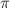and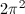, what is the 38th term?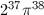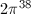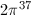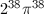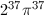Explanation:

The sequence is multiplied by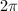each time.

### Example Question #1 : Nth Term Of An Arithmetic Sequence

Find the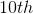term of the following sequence: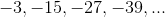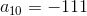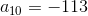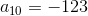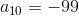Explanation:

The formula for finding the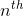term of an arithmetic sequence is as follows: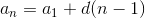where= the difference between consecutive terms= the number of terms

Therefore, to find theterm: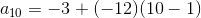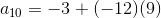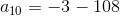### Example Question #9 : Arithmetic Sequences

What is the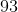rd term of the following sequence: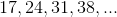?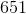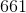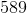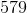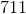Explanation:

Notice that between each of these numbers, there is a difference of; however the first number is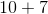, the second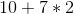, and so forth. This means that for each element, you know that the value must be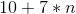, whereis that number's place in the sequence. Thus, for therd element, you know that the value will be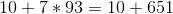or.

### Example Question #10 : Arithmetic Sequences

What is theth term in the following series of numbers:?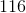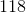148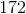Explanation:

Notice that between each of these numbers, there is a difference of. This means that for each element, you will add. The first element is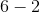or. The second is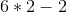or, and so forth... Therefore, for theth element, the value will be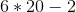or.

### Example Question #2 : Nth Term Of An Arithmetic Sequence

Find the sum of the first fifteen terms in an arithmetic sequence whose sixth term isand whose ninth term is.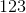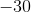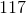Explanation:

Use the formula an = a1 + (n – 1)d

a6 = a1 + 5d

a9 = a1 + 8d

Subtracting these equations yields

a– a9 = –3d

–7 – 8 = –3d

d = 5

a1 = 33

Then use the formula for the series; = –30